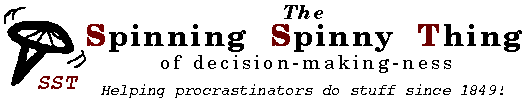## Random number generator

Use this tool to generate random numbers*, for all your randomy needs. Specify whether you want an integer or a decimal fraction, and give the lower and upper bounds for the random number you want. A number will be generated within the range you specify (including both end points).

(*That's pseudo-random, for the geeks out there.)

Your random number will be in this range, including both end points:
Lower:     Upper:
Do you want an integer or a decimal fraction?
Integer     Decimal fraction